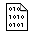## Firefly and PC GAMESS-related discussion clubLearn how to ask questions correctly

Re^3: RPES scan initial coordinates

Pavel Strashnov
paulvstrashnov@mail.ru

Hi Sei,

you can overcome the problem by using DLCs.

\$ZMAT dlc=.t. auto=.t. scan=.t. autofv=.t. IFZMAT(1)=3,10,3,2,4 \$END
and delete vect1(24)=1 from \$surf group.

Also you may want to add
NONVDW(1)=2,4
hbond=0
ifdmod=2
to \$ZMAT to improve your results.
Please find test job results attached.

On Thu Dec 9 '10 6:31am, sei higahsiya wrote
--------------------------------------------
>Hi Pavel,

>Thank you for your interest. It is as followed.

> \$CONTRL SCFTYP=RHF RUNTYP=RSURFACE EXETYP=RUN MAXIT=100 MULT=1 NZVAR=45
> COORD=ZMT \$END
> \$SYSTEM TIMLIM=31536000 MEMORY=40000000 \$END
> \$BASIS GBASIS=N31 NGAUSS=6 NDFUNC=1 NPFUNC=1 DIFFSP=.TRUE. DIFFS=.TRUE. \$END
> \$GUESS GUESS=huckel \$END
> \$statpt opttol=1d-5 nstep=1000 IFREEZ(1)=24 \$end
> \$SCF DIRSCF=.TRUE. \$END
> \$surf ndisp1=37 disp1=5 vect1(24)=1 orig1=0 \$end
> \$DATA
>Title
>C1
>C
>C  1    1.52160
>C   2    1.53410  1 113.3733
>S   2    1.88637  1 112.6257  3 a1
>F   4    1.63249  2  94.5293  1 a2
>F   4    1.61622  2  90.6081  1 a3
>F   4    1.59735  2 176.9384  1 a4
>F   4    1.61180  2  94.9768  1  a5
>F   4    1.62172  2  90.2264  1 a6
>O   3    1.41096  2 115.1032  4  dx
>H   1    1.09237  2 107.2770  3 a7
>H   1    1.08607  11 107.9406  2 a8
>H   2    1.08902  3 107.4905  1 a9
>H   3    1.09146  10 111.8910  2 a10
>H   10    0.96211  3 108.7031  5 a11
>H   1    1.08691  11 108.0355  12 a12
>H   3    1.09283  14 107.3098  10 a13

>a1=-129.7080
>a2=110.0019
>a3=-69.8579
>a4=-126.2670
>a5=20.5918
>a6=-160.4003
>a7=-61.8292
>a8=-120.6862
>a9=-121.9726
>a10=127.4348
>a11=-14.1589
>a12=-118.3293
>a13=-115.6814
>dx=80
> \$END

>Sei

>On Thu Dec 9 '10 5:02am, Pavel Strashnov wrote
>----------------------------------------------
>>Hi,

>>Pavel.

>>On Thu Dec 9 '10 3:38am, sei higahsiya wrote
>>--------------------------------------------
>>>Hi all,

>>>I am performing relaxed potential energy surface scan using Firefly 71G. The problem is when the step proceeds, the initial coordinates except the frozen one keep coming back to the original input values in the \$data section rather than the relaxed coordinates in the previous step.

>>>Is there any way to use the relaxed coordinates in the previous step as an initial coordinate in the next step?This message contains the 1120 kb attachment [ out2.rar ]Fri Dec 10 '10 2:56amThis message read 937 times